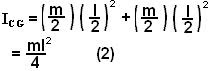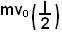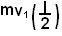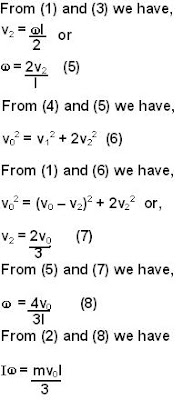## Tuesday, July 1, 2008

### Irodov Problem 1.198After collision two different motions will be induced in the dumbbell, i) a linear velocity v2 with which the entire dumbbell (dumbbell's center of mass) will move and ii) a spin with which the dumbbell will spin about its center of mass, say with an angular velocity w. This is shown in the figure. Suppose that the mass m continues to move with a speed v1 after the collision.

Since this is a completely elastic collision and no external forces or external torques are involved, momentum, angular momentum and energy will be conserved for the entire system.

Conservation of MomentumNote that here we have considered the entire dumbbell as a point mass m concentrated at the center of mass of the dumbbell.

The moment of inertia of the dumbbell about its CG is given by,Conservation of Angular Momentum
Let us consider the angular momentum of the system about the stationary point O, that was collocated with the CG of the dumbbell before collision. The angular momentum of the system before collision is given bywhich is the angular momentum of mass m w.r.t point O. After collision the mass contributes an angular momentum ofsince it moves with a velocity v1. The dumbbell's total angular momentum is the sum of the angular momentum of the CG (due to its linear motion) and the angular momentum of the dumbbell about it CG (due to its spin). Since we have chosen to evaluate the angular momenta about point O which is the initial position of the CG of the dumbbell, the moment arm of the velocity vector of the CG of the dumbbell about O will be 0. Thus the only contribution of the dumbbell's angular momentum comes from its spin about its CG given by Iw. So we have,Conservation of Energy The total energy of the dumbbell after collision is the sum of the kinetic energy of the CG and the kinetic energy due to its spin about the CG. So We have,Now its just a matter of solving (1), (3) and (4) to get w since angular momentum of the dumbbell about its CG is Iw.